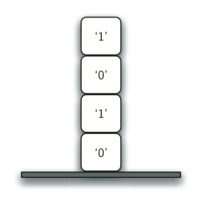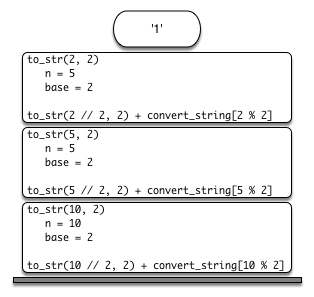# 5.6. Stack Frames: Implementing Recursion¶

Suppose that instead of concatenating the result of the recursive call to `to_str` with the string from `convert_string`, we modified our algorithm to push the strings onto a stack instead of making the recursive call. The code for this modified algorithm is shown in ActiveCode 1.

Each time we make a call to `to_str`, we push a character on the stack. Returning to the previous example we can see that after the fourth call to `to_str` the stack would look like Figure 5. Notice that now we can simply pop the characters off the stack and concatenate them into the final result, `"1010"`.Figure 5: Strings Placed on the Stack During Conversion

The previous example gives us some insight into how Python implements a recursive function call. When a function is called in Python, a stack frame is allocated to handle the local variables of the function. When the function returns, the return value is left on top of the stack for the calling function to access. Figure 6 illustrates the call stack after the return statement on line 4.Figure 6: Call Stack Generated from `to_str(10, 2)`

Notice that the call to `to_str(2 // 2, 2)` leaves a return value of `"1"` on the stack. This return value is then used in place of the function call (`to_str(1, 2)`) in the expression `"1" + convert_string[2 % 2]`, which will leave the string `"10"` on the top of the stack. In this way, the Python call stack takes the place of the stack we used explicitly in Listing 4. In our list summing example, you can think of the return value on the stack taking the place of an accumulator variable.

The stack frames also provide a scope for the variables used by the function. Even though we are calling the same function over and over, each call creates a new scope for the variables that are local to the function.